### Alternating current (AC)

An alternating current is one whose magnitude changes sinusoidally with time. Thus alternating current is given by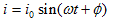Where i0=current amplitude or peak value of alternating current
If T is the time period of alternating current and f is the frequency, then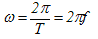Where ω is called angular frequency of A.C and φ is known as phase constant.

Instead of the sine function, AC can also be represented by the cosine function and both representations lead to same results. We will discuss circuits with sine representation of A.C.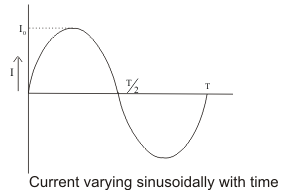Complete set of variations of the current in one-time period T is called cycle.

### Alternating voltage:

The emf or voltage whose magnitude changes sinusoidally with time is known as alternating emf and is represented by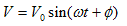where V0 is the peak value of alternating current.

#### Mean Current and RMS Current:

• When an alternating current passed through a moving coil galvanometer it shows no deflection, this is because for one complete cycle mean value of alternating current is zero as AC flows in one direction during the one-half cycle and in opposite direction during another half cycle.
• But mean value of A.C is finite over half cycle.
• So, mean or average value of AC is defined either for positive half cycle or for negative half cycle
• So,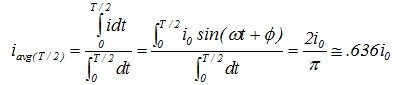• We see that the average value of A.C during the half cycle is .636 times or 63.6% of its peak value.

#### Root mean square (RMS) current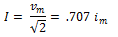#### Mean Voltage and Rms Voltage:

Mean Voltage:Rms Voltage: